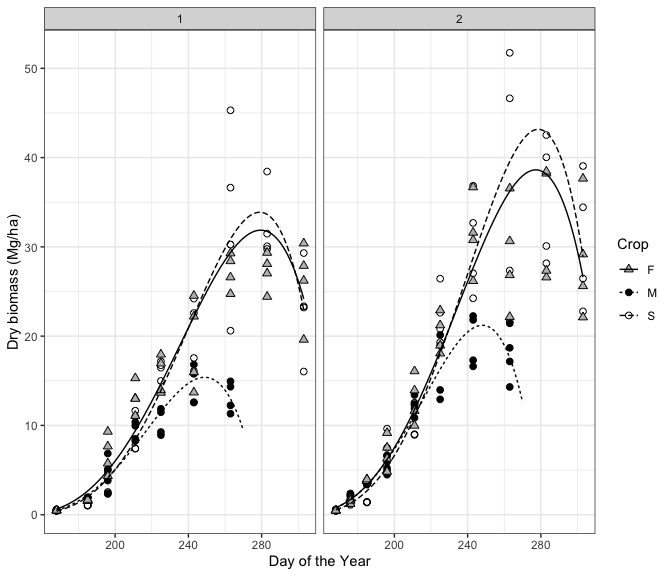# Introduction

The nlraa is distributed as part of publications that illustrates the fit of nonlinear regression models.

## Example

We start by looking at biomass accumulation data from an experiment conducted in Greece by Danalatos and Archontoulis.

data(sm)
str(sm)
## 'data.frame':    236 obs. of  5 variables:
##  $DOY : int 141 141 141 141 141 141 141 141 141 141 ... ##$ Block: int  1 2 3 4 1 2 3 4 1 2 ...
##  $Input: int 2 2 2 2 1 1 1 1 2 2 ... ##$ Crop : Factor w/ 3 levels "F","M","S": 2 2 2 2 2 2 2 2 3 3 ...
##  $Yield: num 0 0 0 0 0 0 0 0 0 0 ... head(sm) ## DOY Block Input Crop Yield ## 1 141 1 2 M 0 ## 2 141 2 2 M 0 ## 3 141 3 2 M 0 ## 4 141 4 2 M 0 ## 5 141 1 1 M 0 ## 6 141 2 1 M 0 The data represents Yield as harvested biomass for three crops: maize (M), fiber sorghum (F) and sweet sorghum (S).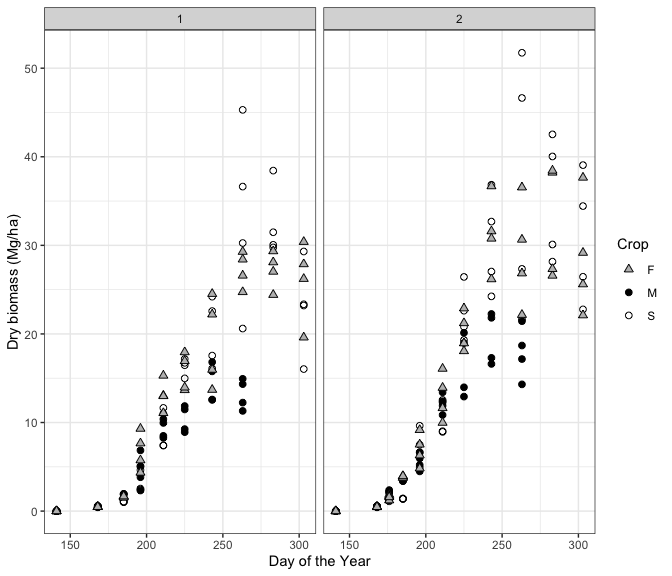Before starting with the model fit we need to manipulate the data by creating an index which describes the experimental unit (eu). We also delete the DOY 141 when crops where planted. sm$eu <- with(sm, factor(Block):factor(Input):factor(Crop))
sm2 <- subset(sm, DOY != 141)

The next step is to create the groupedData which is a convenient structre to be used throughout the fitting process in nlme.

smG <- groupedData(Yield ~ DOY | eu, data = sm2)

Originally, Danalatos et al. (2009) fitted the beta growth function as described by Yin et al. (2003). In nlraa we provide the selfStart function SSbgf to improve the fitting process.

fit.lis <- nlsList(Yield ~ SSbgf(DOY, w.max, t.e, t.m), data = smG)
## Warning: 4 times caught the same error in nls(model, data = data, control =
## controlvals): singular gradient
## But this works better
fit.lis.rp <- nlsList(Yield ~ SSbgrp(DOY, w.max, lt.e, ldt), data = smG) 

There are three crops, two levels of agronomic input and four blocks which results in 24 possible combinations. We fitted the model to these 24 experimental units and obtained apparent convergence in 20 (Note: was only 10 in the original paper, but this improved dramatically when I recomputed the partial derivatives 2020/1/3). Still, this suggests that some modifications are needed.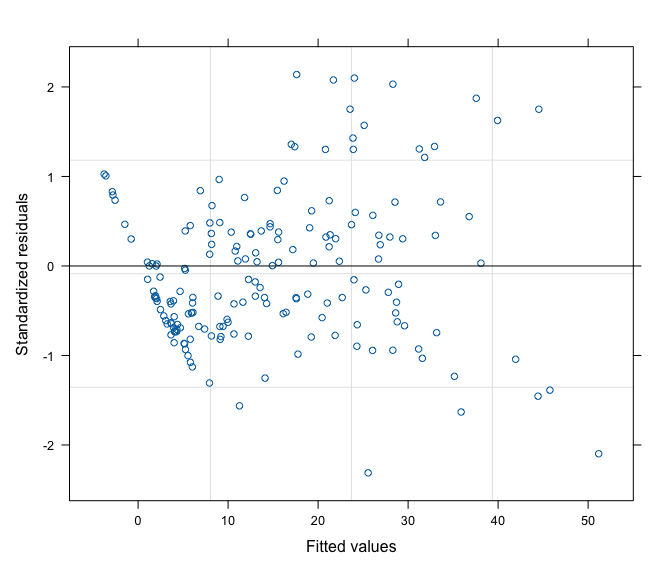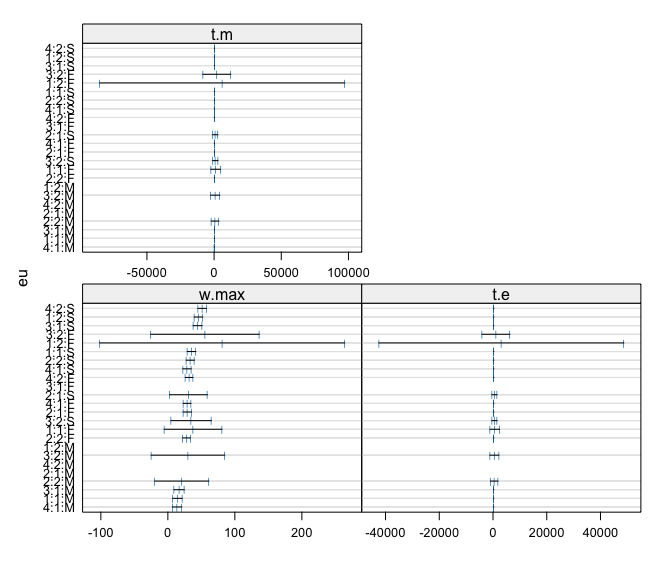From the residuals plot we see some evidence of the inadequacy of the model. In particular the model over predicts at low values. We relax the convergence criteria to achieve a fitted model.

fit.me <- nlme(fit.lis, control = list(maxIter = 100, msMaxIter = 300, pnlsMaxIter = 20))
## Warning in (function (model, data = sys.frame(sys.parent()), fixed, random, :
## Iteration 1, LME step: nlminb() did not converge (code = 1). PORT message:
## function evaluation limit reached without convergence (9)

Despite the message, we do obtain a ‘partially’ fitted model.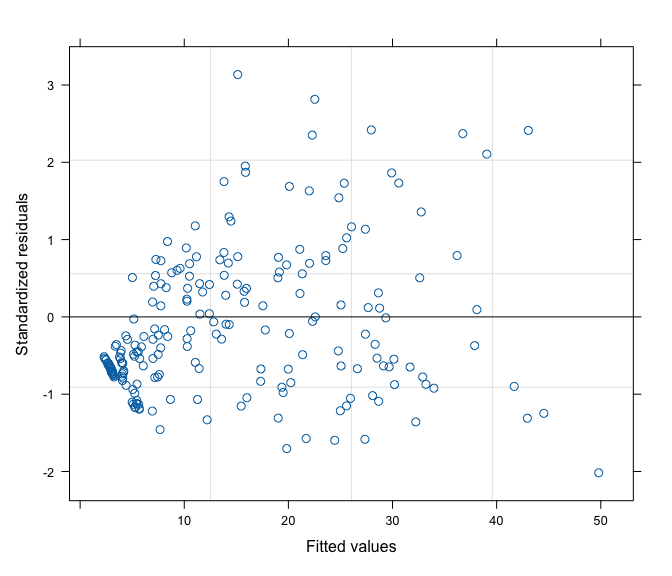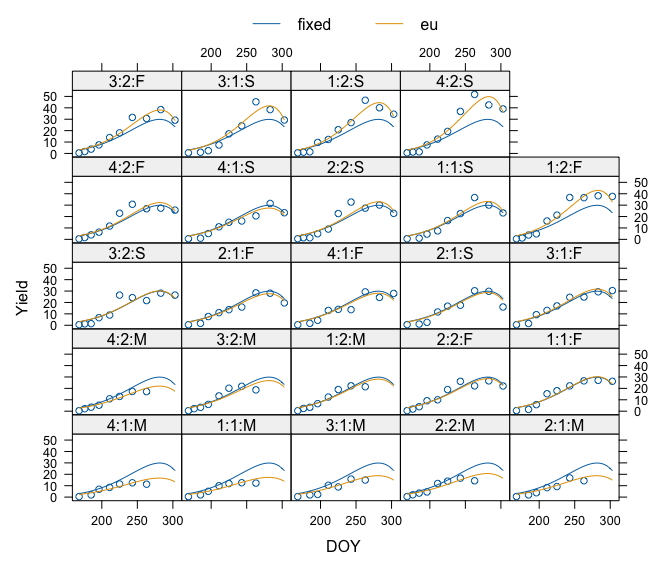A modified beta growth function proposed by Yin et. al (2003) – included in the errata – allows for a delayed start of growth by modifying the $$t_b$$ parameter.

$y = w_b + (w_{max} - w_b) \left (1 + \frac{t_e - t}{t_e - t_m} \right ) \left (\frac{t - t_b}{t_e - t_b} \right )^\frac{t_e - t_b}{t_e - t_m}$

$t_b < t_m < t_e$

We include this as bgf2 but not the selfStart version at this point. We also fix the $$w_b$$ and the $$t_b$$ parameters, so they are not part of the fitting process. There are good reasons for this: We know the initial biomass is minimal (seed weight) and we know the day of planting (it does not need to be optimized).

fit.lis2 <- nlsList(Yield ~ bgf2(DOY, w.max, w.b = 0, t.e, t.m, t.b = 141),
data = smG,
start = c(w.max = 30, t.e=280, t.m=240))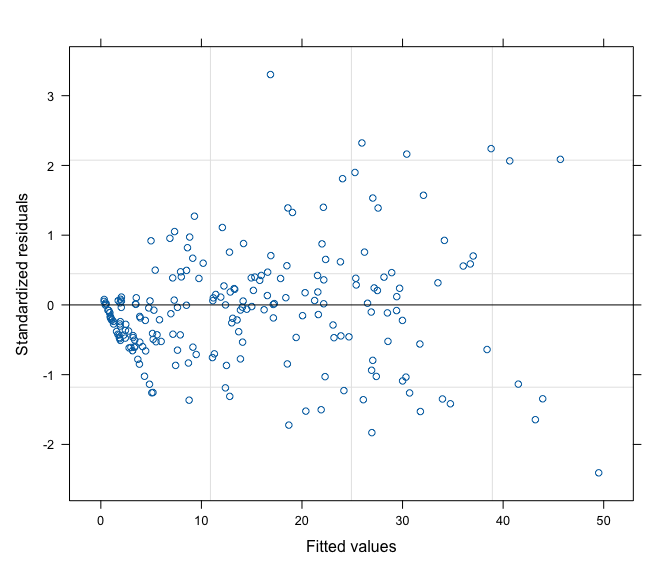The previous figure shows a much lower bias at lower values.

We proceed to fit the non-linear mixed model and then we simplify the variance-covariance random effects structure.

fit.me2 <- nlme(fit.lis2)
## Warning in (function (model, data = sys.frame(sys.parent()), fixed, random, :
## Iteration 2, LME step: nlminb() did not converge (code = 1). Do increase
## 'msMaxIter'!
## Error message, but the next model is the one we care about
fit2.me2 <- update(fit.me2, random = pdDiag(w.max + t.e + t.m ~ 1))
anova(fit.me2, fit2.me2)
##          Model df      AIC      BIC    logLik   Test  L.Ratio p-value
## fit.me2      1 10 1167.754 1201.320 -573.8771
## fit2.me2     2  7 1176.156 1199.652 -581.0778 1 vs 2 14.40146  0.0024
## The second model is simpler and it seems to be marginally better than
## the orginial, but we need to keep in mind that the simpler model
## converges much more easily

Some of the covariances might be significant, but we’ll look at this later. We will next include the effects of Crop type and Input in the fixed part of the model. We want to know how the parameters are affected by the treatment effects.

fe <- fixef(fit2.me2) ## Some starting values with visual help
fit3.me2 <- update(fit2.me2, fixed = list(w.max + t.e + t.m ~ Crop),
start = c(fe, -10, 20, fe, -40, 0, fe, -40, 0))
## We next include the Input
fe2 <- fixef(fit3.me2)
fit4.me2 <- update(fit3.me2, fixed = list(w.max + t.e + t.m
~ Crop + Input),
start = c(fe2[1:3], 0, fe2[4:6], 0, fe2[7:9], 0))
## and the interaction
fe3 <- fixef(fit4.me2)
fit5.me2 <- update(fit4.me2,
fixed = list(w.max + t.e + t.m
~ Crop + Input + Crop:Input),
start = c(fe3[1:4], 0, 0,
fe3[5:8], 0, 0,
fe3[9:12], 0, 0))

The current model displays some evidence of unequal variance as shown in the figure. The amount of dispersion around zero is smaller for low fitted values and the amount for large fitted values is larger.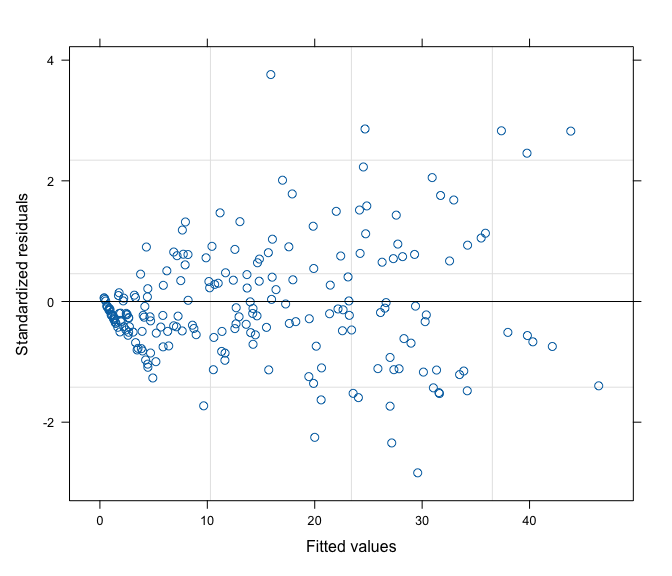We fit two models one where the variance depends on the Crop (since visually the crops are so different) and another one where it does not depend on the Crop.

fit6.me2 <- update(fit5.me2,
weights = varPower(form = ~ fitted(.) | Crop))

fit7.me2 <- update(fit6.me2, weights = varPower(form = ~ fitted(.)))
## Warning in (function (model, data = sys.frame(sys.parent()), fixed, random, :
## Iteration 1, LME step: nlminb() did not converge (code = 1). PORT message:
## false convergence (8)
anova(fit6.me2, fit7.me2)
##          Model df      AIC      BIC    logLik   Test  L.Ratio p-value
## fit6.me2     1 25 934.4841 1018.399 -442.2421
## fit7.me2     2 23 940.5031 1017.705 -447.2515 1 vs 2 10.01896  0.0067

Model fit6.me2 is better according to the AIC criteria and the likelihood ratio test.

fit6.me2
## Nonlinear mixed-effects model fit by maximum likelihood
##   Model: Yield ~ bgf2(DOY, w.max, w.b = 0, t.e, t.m, t.b = 141)
##   Data: smG
##   Log-likelihood: -442.2421
##   Fixed: list(w.max + t.e + t.m ~ Crop + Input + Crop:Input)
## w.max.(Intercept)       w.max.CropM       w.max.CropS       w.max.Input
##       25.11412909      -15.54706291       -0.52866804        6.75566294
## w.max.CropM:Input w.max.CropS:Input   t.e.(Intercept)         t.e.CropM
##       -0.93045587        2.53649158      281.72491203      -32.06305436
##         t.e.CropS         t.e.Input   t.e.CropM:Input   t.e.CropS:Input
##       -2.32139922       -2.25004795        1.43274745        1.84876033
##   t.m.(Intercept)         t.m.CropM         t.m.CropS         t.m.Input
##      237.08075618      -18.66441608        3.57254905       -1.26661255
##   t.m.CropM:Input   t.m.CropS:Input
##       -0.09073866        0.66439683
##
## Random effects:
##  Formula: list(w.max ~ 1, t.e ~ 1, t.m ~ 1)
##  Level: eu
##  Structure: Diagonal
##         w.max.(Intercept) t.e.(Intercept) t.m.(Intercept)  Residual
## StdDev:      2.258637e-08     0.001750977     1.51305e-07 0.3479004
##
## Variance function:
##  Structure: Power of variance covariate, different strata
##  Formula: ~fitted(.) | Crop
##  Parameter estimates:
##         M         F         S
## 0.7027792 0.8586347 0.8960404
## Number of Observations: 212
## Number of Groups: 24

Since random effects are almost zero. We remove them from the model and use the gnls function which is specifically written for models without random effects.

## Random effects are almost zero
fit8.me2 <- gnls(Yield ~ bgf2(DOY, w.max, t.e, t.m, w.b=0, t.b=141),
data = smG,
params = list(w.max + t.e + t.m ~ Crop + Input
+ Crop:Input),
weights = varPower(form = ~ fitted(.) | Crop),
start = fixef(fit7.me2))
anova(fit6.me2, fit8.me2)
##          Model df      AIC      BIC    logLik   Test      L.Ratio p-value
## fit6.me2     1 25 934.4841 1018.399 -442.2421
## fit8.me2     2 22 928.4834 1002.328 -442.2417 1 vs 2 0.0006862451       1

Model fit8.me2 is better than fit6.me2 according to AIC and BIC.

anova(fit8.me2)
## Denom. DF: 194
##                   numDF   F-value p-value
## w.max.(Intercept)     1  12677.85  <.0001
## w.max.Crop            2    985.63  <.0001
## w.max.Input           1    482.00  <.0001
## w.max.Crop:Input      2     36.67  <.0001
## t.e.(Intercept)       1  26077.38  <.0001
## t.e.Crop              2     97.90  <.0001
## t.e.Input             1     35.98  <.0001
## t.e.Crop:Input        2     64.25  <.0001
## t.m.(Intercept)       1 139814.54  <.0001
## t.m.Crop              2    151.45  <.0001
## t.m.Input             1      0.77  0.3808
## t.m.Crop:Input        2      0.04  0.9636

This shows that the Crop, Input and interaction are significant for all terms except for the t.m parameter.

Residuals look good with much less overprediction at lower values. The autocorrelation does not appear to be a concern (not shown).

print(plot(fit8.me2))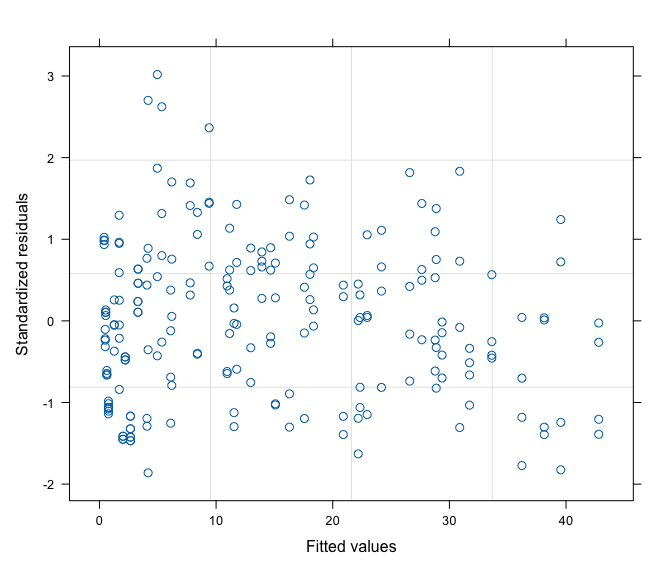We finalize the fitting exercise by plotting observed and predicted values.

smG$prds <- fitted(fit8.me2) doys <- 168:303 ndat <- expand.grid(DOY=doys, Crop= unique(smG$Crop), Input=c(1,2))
ndat$preds <- predict(fit8.me2, newdata = ndat) ## Here I'm just removing prediction for maize that go beyond ## day of the year 270 ndat2 <- ndat ndat2[ndat2$Crop == "M" & ndat2\$DOY > 270,"preds"] <- NA
ndat2 <- na.omit(ndat2)